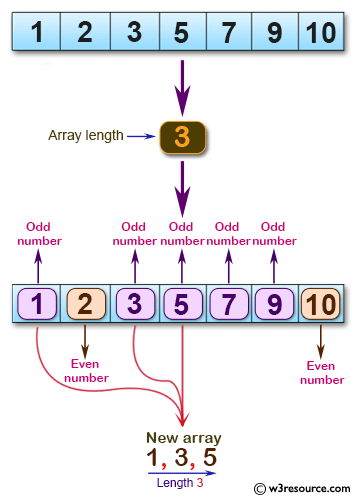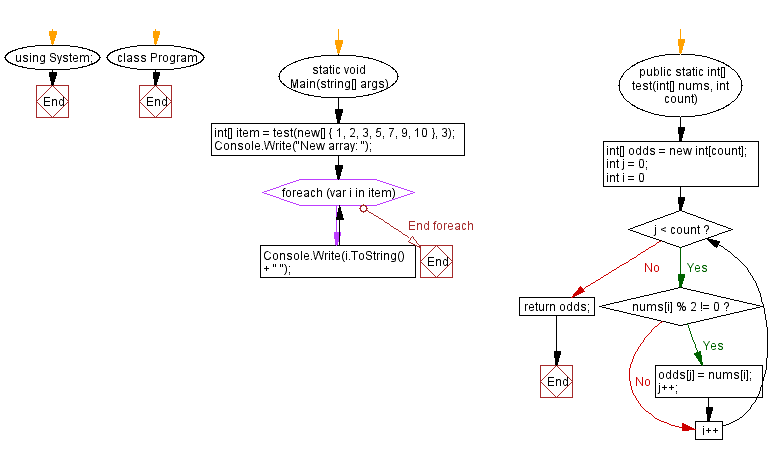﻿ C# - Create an array of odd numbers from a array of integers# C# Sharp Basic Algorithm Exercises: Create a new array of given length using the odd numbers from a given array of positive integers

## C# Sharp Basic Algorithm: Exercise-140 with Solution

Write a C# Sharp program to create a new array of given length using the odd numbers from a given array of positive integers.

Pictorial Presentation:Sample Solution:

C# Sharp Code:

``````using System;

namespace exercises
{
class Program
{
static void Main(string[] args)
{

int[] item = test(new[] {1,2,3,5,7,9,10},3);
Console.Write("New array: ");
foreach(var i in item)
{
Console.Write(i.ToString()+" ");
}
}
public static int[] test(int[] nums, int count)
{
int[] evens = new int[count];
int j = 0;

for (int i = 0; j < count; i++)
{
if (nums[i] % 2 != 0)
{
evens[j] = nums[i];
j++;
}
}

return evens;
}

}
}
```
```

Sample Output:

`New array: 1 3 5`

Flowchart:C# Sharp Code Editor:

Improve this sample solution and post your code through Disqus

What is the difficulty level of this exercise?

Test your Programming skills with w3resource's quiz.

﻿